## SMARTCROP

 Automatically crops an image to a given size around the hightest detail region.

 USAGE: smartcrop -w width -h height [-m metric] [-s search] [-l lowerthresh] [-u upperthresh] [-f factor] [-e enlarge] [-g graymode] [-b blur] [-n normalize] infile outfile USAGE: smartcrop [-help] -w ... width ......... desired crop width; integer>0; required -h ... height ........ desired crop height; integer>0; required -m ... metric ........ metric to use to process the image for detail; choices are ...................... std (for standard_deviation), sobel (for sobel type grayscale ...................... edges), canny (for canny type binary edges) and ...................... corners (for morphologic corner detection); default=canny; -s ... search ........ search technique; choices are basic (slow), pyramid (fast) and ...................... fft (fast); default=basic -d ... dimension ..... radius for metric=std and sigma for metric=canny; integer>0 ...................... for std and float>0 for canny; default=1. -l ... lowerthresh ... lower threshold for canny edges (percent); smaller is more edges; ...................... 0<=integer<=100; default=10 -u ... upperthresh ... upper threshold for canny edges (percent); smaller is more edges; ...................... 0<=integer<=100; default=30 -f ... factor ........ scale factor for size reduction for search=pyramid; integer>0; ...................... default=5 -e ... enlarge ....... enlarge full resolution search area all around in pixels for ...................... search=pyramid; integer>0; default=20 -g ... graymode ...... mode of converting to grayscale; grayscale or maximum; ...................... default=grayscale -b ... blur .......... blur the detail image before normalizing; integer>=0; zero ...................... means no blur; default=20 -n ... normalize ..... normalize the detail image before cropping; equalize or ...................... autolevel; default=equalize PURPOSE: To automatically crop an image to a given size around the hightest detail region. DESCRIPTION: SMARTCROP automatically crops an image to a given size around the hightest detail region. It tries to find the best region of the given size to crop based upon fundamental type details in the image. The detail metric can be: standard deviation, sobel grayscale edges or canny binary edges. The region will be the one with the largest average grayscale. This is a low to medium intelligent method in that it uses fundamental measures of detail. So perhaps it should really be called a semi-intelligent method. It does not try to do face detection. The method of locating the best match uses rmse subimage search. For search=basic, it uses compare at full resolution. For search=pyramid, it uses a coarse to fine 2-level pyramid using compare. For search=fft, it does a compare equivalent on the full resolution image using FFT processing via my script, rmsecorr. Results will depend upon the size of the crop dimensions desired and the metric and search methods. The script does best with a single region of high detail and the rest of the image low detail. Too much detail in the image or too small width and or height may give totally irrelevant results to what the user wants. ARGUMENTS: -w width ... WIDTH is the desired crop width. Values are integers>0. This is a required argument. -h height ... HEIGHT is the desired crop height. Values are integers>0. This is a required argument. -m metric ... METRIC to use to process the image for detail. The choices are std (for standard_deviation), sobel (for sobel type grayscale edges), canny (for canny type binary edges) and corners (for morphologic corner detection). The default=canny. -s search ... SEARCH technique. The choices are basic (slow), pyramid (fast) and fft (fast). The default=basic. -d dimension ... DIMENSIONS is either the radius for metric=std or sigma for metric=canny. Values are integers>0 for std and floats>0 for canny. The default=1. -l lowerthresh LOWERTHRESH is the lower threshold for canny edges (in percent). Smaller values produce more edges. Values are 0<=integers<=100. The default=10. -u upper UPPERTHRESH is the upper threshold for canny edges (in percent). Smaller values produce more edges. Values are 0<=integers<=100. The default=30. -f factor ... FACTOR is the scale factor for size reduction for search=pyramid. Values are integers>0. The default=5. -e enlarge ... ENLARGE full resolution search area all around in pixels for search=pyramid. Values are integers>0. The default=20. -g graymode ... GRAYMODE is the mode of converting the detail image to grayscale. The choices are: grayscale (change colorspace to gray) or maximum (separate channels and take the maximum of the channels at each pixels). The default=maximum. Usually maximum is better than grayscale. But occasionally the opposite is true. -b blur ... BLUR the detail image before normalizing. Values are integers>=0. A value of zero means no blur. Typical values are either 0 or between 20 and 30. The default=20. Usually blurring is better than not. But occasionally the opposite is -n normalize ... NORMALIZE the detail image before cropping. The choices are: equalize or autolevel. The default=equalize. Usually equalizing is better than autolevel. But occasionally the opposite is true. REQUIREMENTS: Requires IM 6.6.9.1 or higher for metric=std. Requires IM 6.8.9.0 or higher for metric=canny. But due to a bug in IM 7 for the -canny operator, results will not be correct until IM 7.0.6.1 or higher, when the bug was fixed. Requires IM 6.5.9.3 or higher for metric=corners. Requires IM 6.6.1.8 or higher for metric=sobel. CAVEAT: No guarantee that this script will work on all platforms, nor that trapping of inconsistent parameters is complete and foolproof. Use At Your Own Risk.

### EXAMPLES

 Example 1 Original (original)Arguments: -m canny -s basic -g maximum -n equalize -b 20 Arguments: -m corners -s basic -g maximum -n equalize -b 20 Arguments -m sobel -s basic -g maximum -n equalize -b 20 Arguments -m std -s basic -g maximum -n equalize -b 20Arguments: -m canny -s basic -g maximum -n equalize -b 0 Arguments: -m corners -s basic -g maximum -n equalize -b 0 Arguments -m sobel -s basic -g maximum -n equalize -b 0 Arguments -m std -s basic -g maximum -n equalize -b 0Example 2 Original (original)Arguments: -m canny -s basic -g maximum -n equalize -b 20 Arguments: -m corners -s basic -g maximum -n equalize -b 20 Arguments -m sobel -s basic -g maximum -n equalize -b 20 Arguments -m std -s basic -g maximum -n equalize -b 20Arguments: -m canny -s basic -g maximum -n equalize -b 0 Arguments: -m corners -s basic -g maximum -n equalize -b 0 Arguments -m sobel -s basic -g maximum -n equalize -b 0 Arguments -m std -s basic -g maximum -n equalize -b 0Example 3 Original (original)Arguments: -m canny -s basic -g maximum -n equalize -b 20 Arguments: -m corners -s basic -g maximum -n equalize -b 20 Arguments -m sobel -s basic -g maximum -n equalize -b 20 Arguments -m std -s basic -g maximum -n equalize -b 20Arguments: -m canny -s basic -g maximum -n equalize -b 0 Arguments: -m corners -s basic -g maximum -n equalize -b 0 Arguments -m sobel -s basic -g maximum -n equalize -b 0 Arguments -m std -s basic -g maximum -n equalize -b 0Example 4 Original (original)Arguments: -m canny -s basic -g maximum -n equalize -b 20 Arguments: -m corners -s basic -g maximum -n equalize -b 20 Arguments -m sobel -s basic -g maximum -n equalize -b 20 Arguments -m std -s basic -g maximum -n equalize -b 20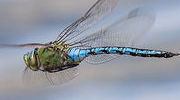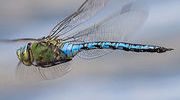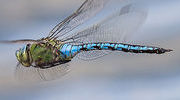Arguments: -m canny -s basic -g maximum -n equalize -b 0 Arguments: -m corners -s basic -g maximum -n equalize -b 0 Arguments -m sobel -s basic -g maximum -n equalize -b 0 Arguments -m std -s basic -g maximum -n equalize -b 0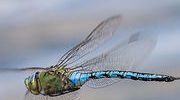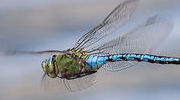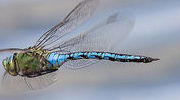Example 5 -- Basic vs. Pyramid vs. FFT Original (original)Arguments: -m canny -s basic -g maximum -n equalize -b 20 time: 10.5 sec Arguments: -m canny -s pyramid -g maximum -n equalize -b 20 time: 0.8 sec Arguments -m canny -s fft -g maximum -n equalize -b 20 time: 2.9 secWhat the script does is as follows for metric=canny and search=basic: Applies a local standard deviation process to the input and convert to grayscale Does a compare of the processed image against a white image of the desired subsection size Extracts the crop offsets using the best match coordinates Crops the input This is equivalent to the following IM commands if [ "\$im_version" -ge "07000000" ]; then preface="magick" else preface="" fi convert "\$infile" -canny 0x1+\${lowerthresh}%+\${upperthresh}% -separate \ -evaluate-sequence max -blur 0x\$blur -equalize \$dir/tmpI2.mpc coords=`\$preface compare -metric rmse -subimage-search -dissimilarity-threshold 1 \ \$dir/tmpI2.mpc \( -size \${width}x\${height} xc:white \) null: 2>&1 | cut -d\ -f4` xoff=`echo "\$coords" | cut -d, -f1` yoff=`echo "\$coords" | cut -d, -f2` convert \$dir/tmpI1.mpc -crop \${width}x\${height}+\${xoff}+\${yoff} +repage "\$outfile"